# Arcs and Subtended Angles

Arcs and Subtended Angles
Go back to  'Circles'

 1 How to find the Angle Subtended by an Arc? 2 Angle Subtended by an Arc at the Centre 3 Angles in the Same Segment of a Circle are Equal 4 Thinking out of the Box! 5 Solved Examples on Angle Subtended by an Arc 6 Important Notes on Angle Subtended by an Arc 7 Practice Question on Angle Subtended by an Arc 8 Maths Olympiad Sample Papers 9 Frequently Asked Questions (FAQs)

We at Cuemath believe that Math is a life skill. Our Math Experts focus on the “Why” behind the “What.” Students can explore from a huge range of interactive worksheets, visuals, simulations, practice tests, and more to understand a concept in depth.

Book a FREE trial class today! and experience Cuemath's LIVE Online Class with your child.

## How do you Find the Angle Subtended by an Arc in Circle?

An arc of a circle is any part of the circumference.

The angle subtended by an arc at any point is the angle formed between the two line segments joining that point to the end-points of the arc.

In the following figure, an arc of the circle shown subtends an angle $$\alpha$$ at a point on the circumference, and an angle $$\beta$$ at the centre $$O$$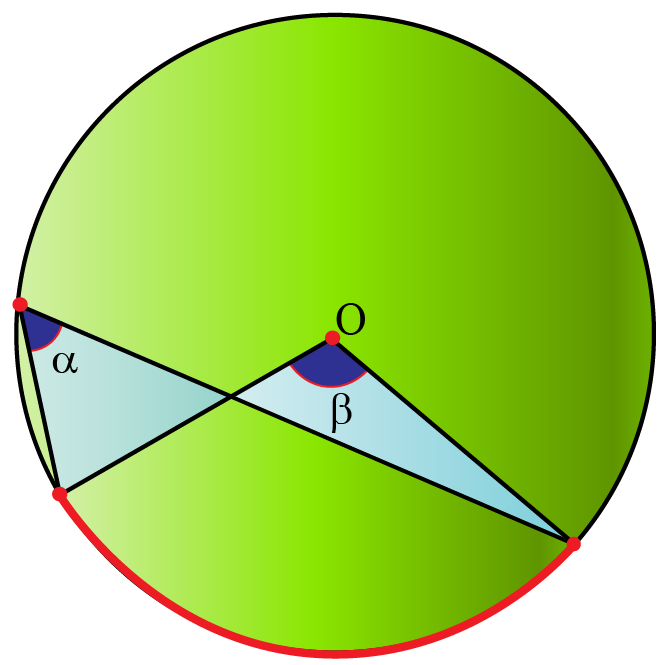We are interested in the relation between the angle which a given arc subtends anywhere on the circumference and the angle which that arc subtends at the circle’s centre.

The relation between them is simple, and extremely important.

## Angle Subtended by an Arc at the Centre

### Theorem:

The angle subtended by an arc of a circle at its centre is twice of the angle it subtends anywhere on the circle’s circumference.

The proof of this theorem is quite simple, and uses the exterior angle theorem – an exterior angle of a triangle is equal to the sum of the opposite interior angles.

If the two opposite interior angles happen to be equal, then the exterior angle will be twice of any of the opposite interior angles.

Proof:

Consider the following figure, in which an arc (or segment) $$AB$$ subtends $$\angle AOB$$ at the centre $$O$$, and $$\angle ACB$$ at a point $$C$$ on the circumference.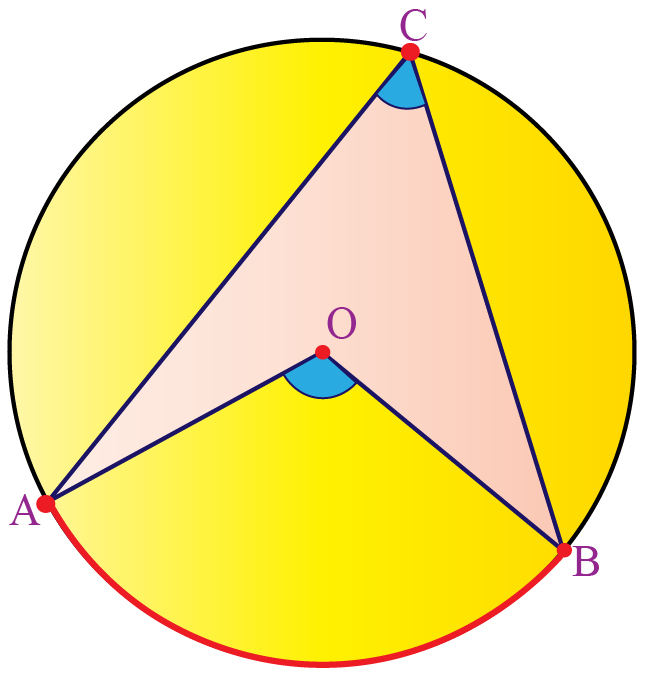We have to prove that $$\angle AOB = 2 \times \angle ACB$$. Draw the line through $$O$$ and $$C$$, and let it intersect the circle again at $$D$$, as shown.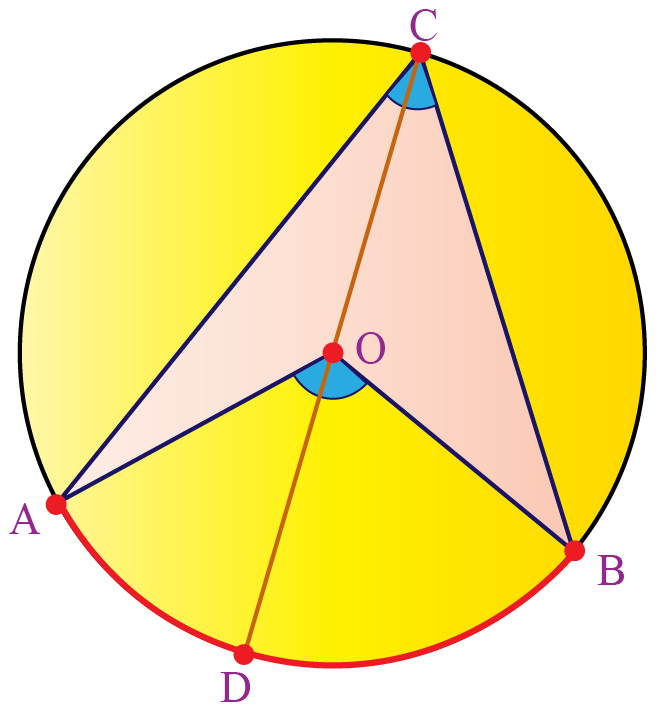We make the following observations.

1. In $$\Delta OAC$$, $$\angle OAC = \angle OCA$$, because $$OA = OC$$

2. In $$\Delta OBC$$, $$\angle OBC = \angle OCB$$, because $$OB = OC$$

Hence,

$$\angle AOD = 2 \times \angle ACO \;\;\;\; \cdots (1)$$

$$\angle DOB = 2 \times \angle OCB \;\;\;\; \cdots (2)$$

Add equations $$(1)$$ and $$(2)$$:

$$\angle AOD + \angle DOB = 2 \times (\angle ACO + \angle OCB)$$

$$\implies \angle AOB = 2 \times \angle ACB$$

This completes the proof of the theorem.

You can experiement with the simulation below to understand the relationship between the angles subtended by an arc at the centre and at a point on the circle.

What if arc $$AB$$ is such that it subtends a reflex angle at the centre?

Well, our proof doesn’t change, as the following figure shows.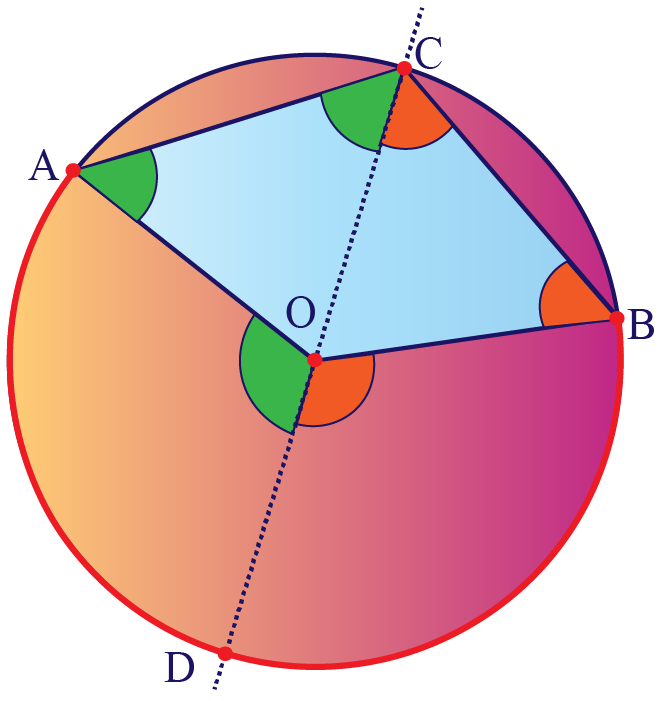You can now apply exactly the same steps as we did earlier, and arrive at the same result: $$\angle AOB = 2 \times \angle ACB$$

Another configuration would be when $$O$$ does not lie within $$\angle ACB$$

Will the result still hold, as in the following figure?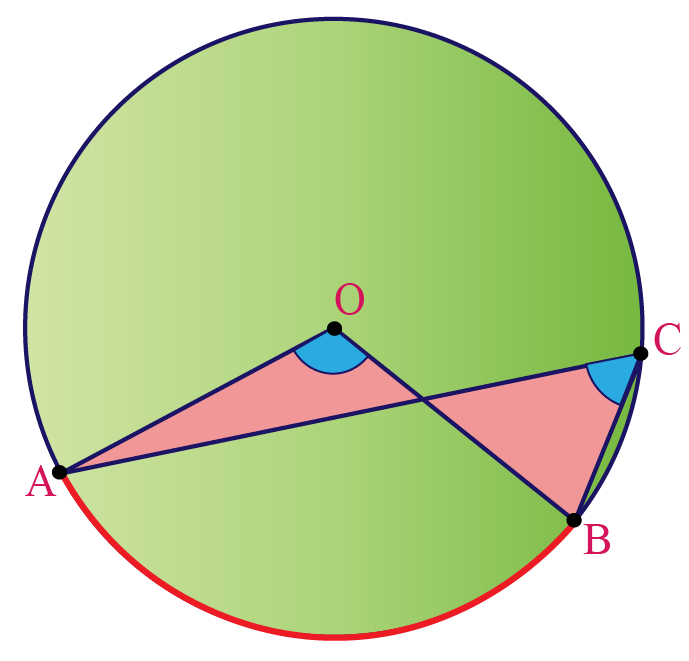Yes, it will.

Join $$C$$ to $$O$$ again and extend it to $$D$$ on the circumference of the circle.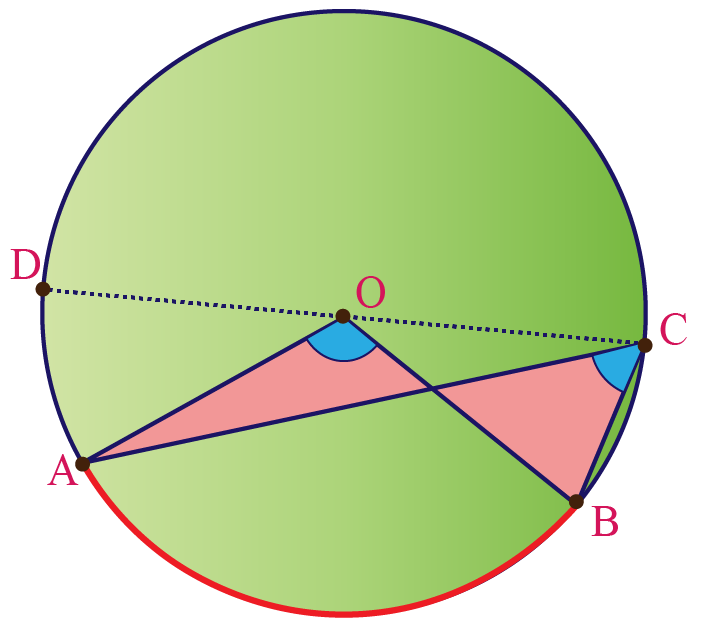Use the Exterior Angle Theorem again and note that,

1. $$\angle BOD = 2 \times \angle BCD \;\;\;\; \cdots (1)$$

2. $$\angle AOD = 2 \times \angle ACD \;\;\;\; \cdots (2)$$

To avoid cluttering, these angles have not been highlighted in the figure, but make sure you observe them carefully.

Subtract Equation $$(2)$$ from $$(1)$$

$$\angle BOD - \angle AOD = 2 \times (\angle BCD - \angle ACD)$$

$$\implies \angle AOB = 2 \times \angle ACB$$

This theorem leads to an interesting corollary, which is discussed next.

### Corollary:

The angle in a semi-circle is a right angle.

Consider the figure below, where $$AB$$ is a diameter of the circle.

We need to prove that $$\angle ACB = 90^{\circ}$$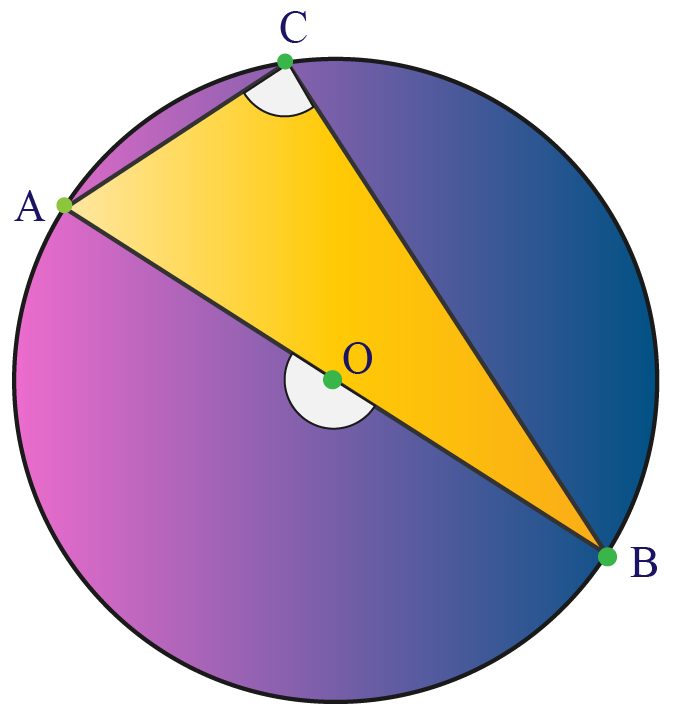Proof:

Since $$\angle AOB =180^{\circ}$$,

\angle ACB = \begin{align}\frac{1}{2}\end{align} \times \angle AOB = 90^{\circ}

This theorem also leads to the following result.

## Angles in the Same Segment of a Circle are Equal

### Theorem:

Angles in the same segment of a circle are equal.

In other words, an arc in a circle will subtend equal angles anywhere on the circumference.

Consider the following figure, which shows an arc $$AB$$ subtending angles $$ACB$$ and $$ADB$$ at two arbitrary points $$C$$ and $$D$$ on the circumference.

$$O$$ is the centre of the circle.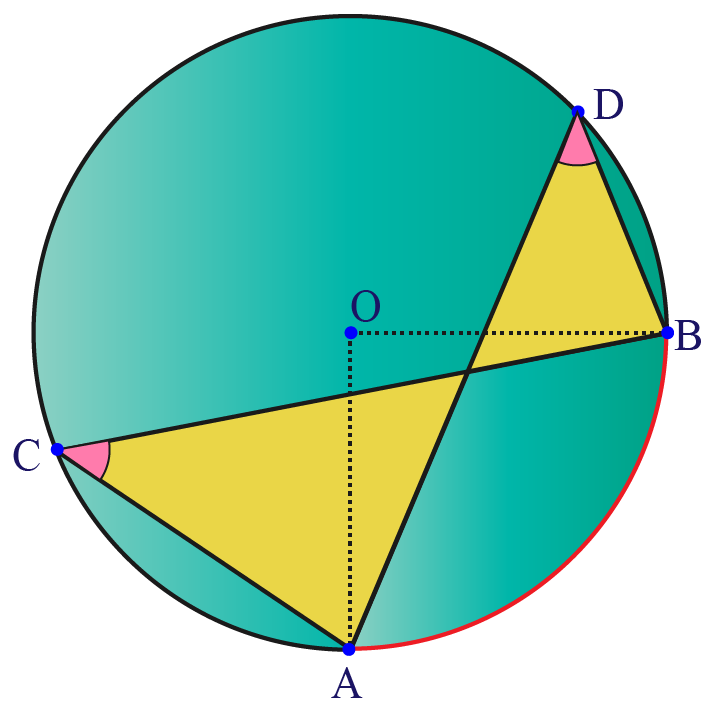We need to prove that $$\angle ACB = \angle ADB$$

Proof:

Using our previous theorem, we have that

\angle ACB = \begin{align}\frac{1}{2}\end{align} \times \angle AOB \;\;\;\; \cdots (1)

\angle ADB= \begin{align}\frac{1}{2}\end{align} \times \angle AOB \;\;\;\; \cdots (2)

From equations $$(1)$$ and $$(2)$$, we get

$$\angle ACB = \angle ADB$$

Now you can experiment with the simulation below to examine the relationship.Think Tank
1. If the angles subtended by the chords of a circle at a centre are equal, are the chords equal?
2. The chords of congruent circles subtend equal  angles at their centres. Are the chords equal?

Help your child score higher with Cuemath’s proprietary FREE Diagnostic Test. Get access to detailed reports, customized learning plans, and a FREE counseling session. Attempt the test now.

## Solved Examples

 Example 1

Find the value of $$x$$ (in degrees) in each of the following figures (wherever indicated, $$O$$ is the centre of the circle)

I.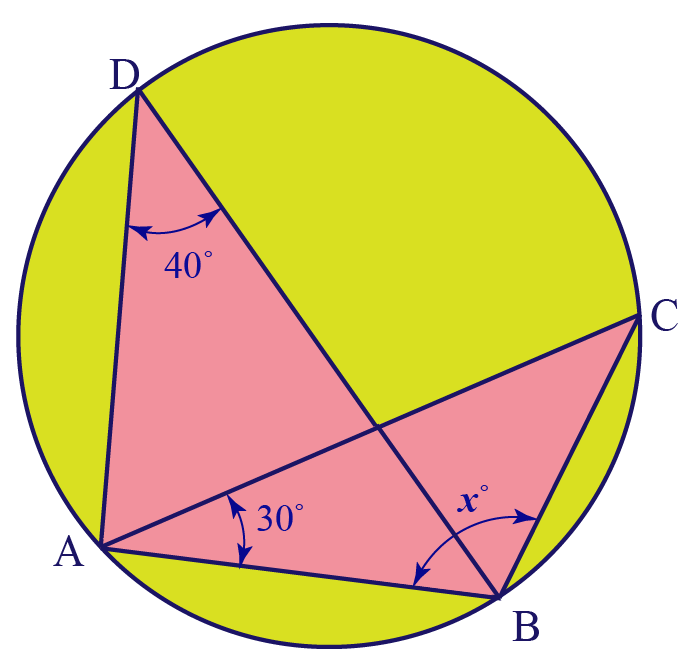Solution:

From the figure, we can say that $$\angle ACB = \angle ADB = 40^{\circ}$$

Using the angle sum property in $$\Delta ACB$$,

\begin{align}\angle BAC + \angle ACB + x^{\circ} &=180^{\circ}\\ 30^{\circ} + 40^{\circ} + x^{\circ}&=180^{\circ}\\x^{\circ}=110^{\circ}\end{align}

 $$\therefore$$ The value of $$x$$ is 110

II.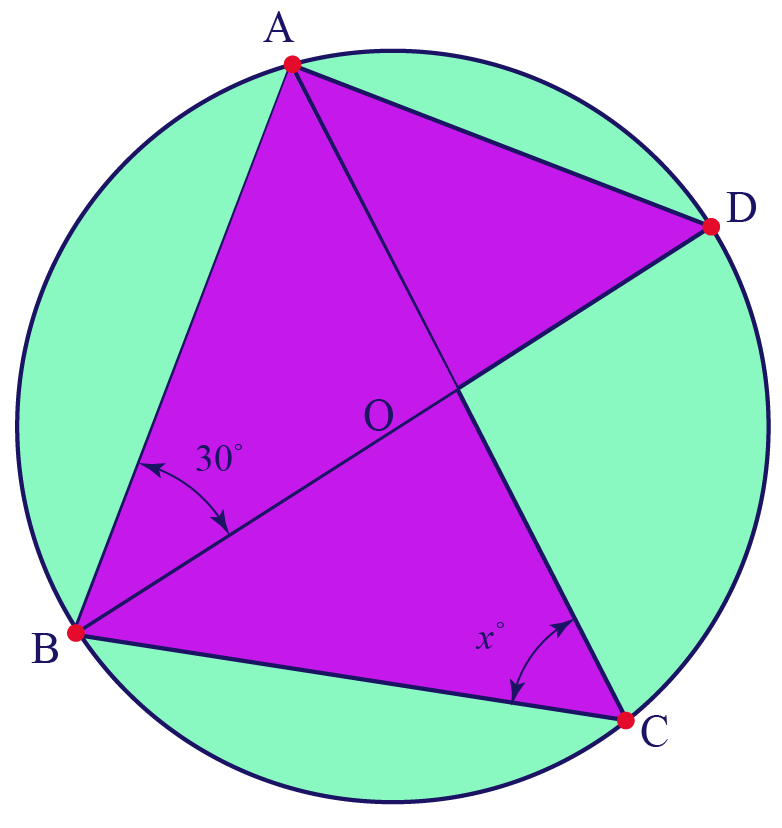Solution:

$$\angle BAD$$ is an angle in a semi-circle, and is therefore, a right angle.

Using the Angle Sum Property in $$\Delta ABD$$, we have:

\begin{align}\angle ADB&=180^{\circ}-(30^{\circ}+90^{\circ})\\&=60^{\circ}\end{align}

Thus, $$\angle ACB=\angle ADB=60^{\circ}$$

 $$\therefore$$ The value of $$x$$ is 60

III.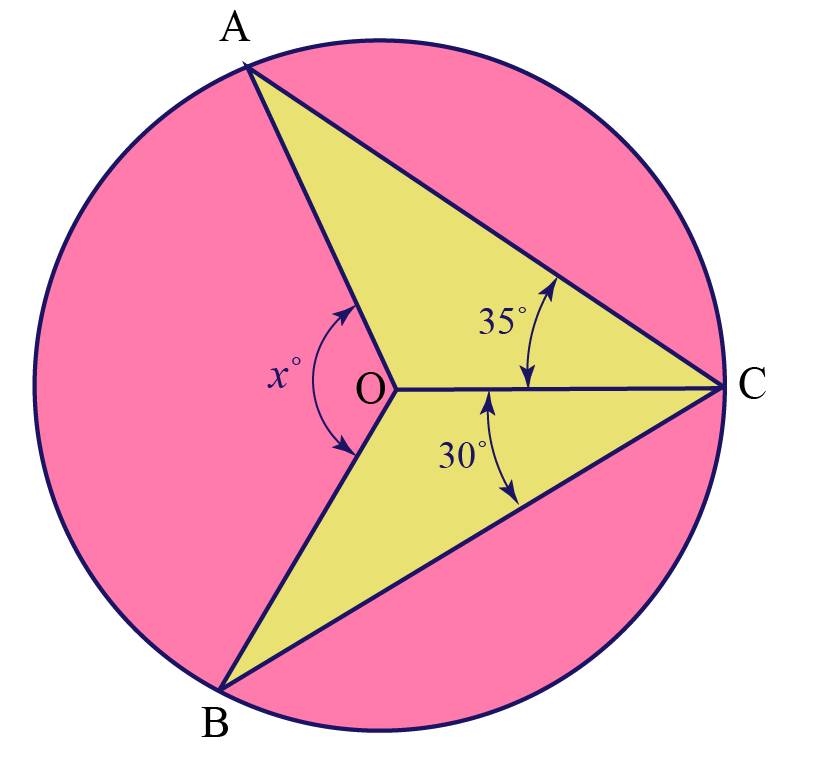Solution:

We have $$\angle AOB=2\angle ACB$$

So,

\begin{align}\angle AOB &= 2\angle ACB\\ &=2(35^{\circ}+30^{\circ})\\ &=130^{\circ}\end{align}

 $$\therefore$$ The value of $$x$$ is 130

IV.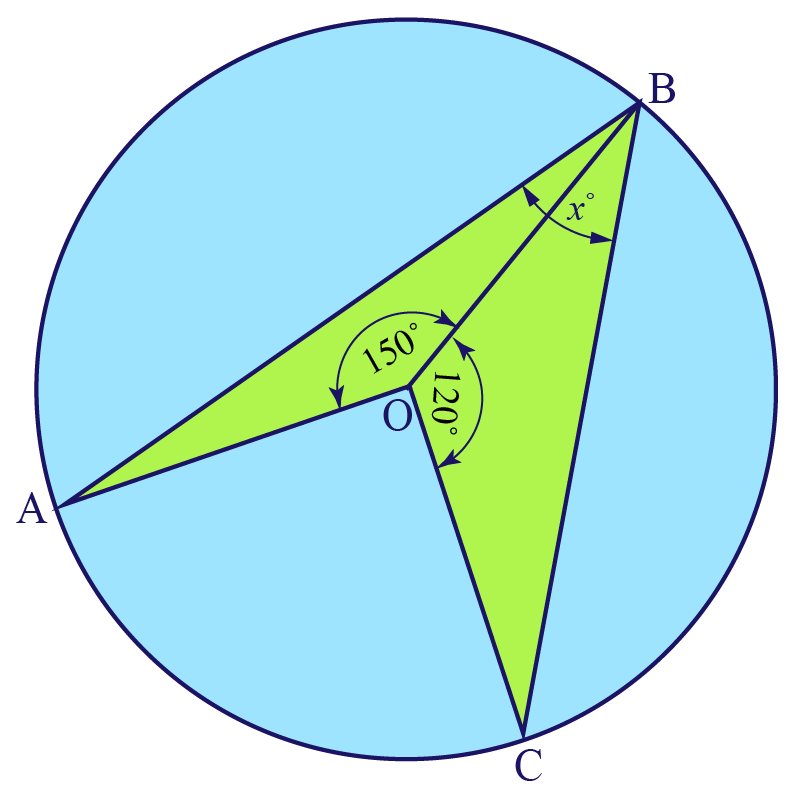Solution:

We note that,

\begin{align} \angle AOC&=360^{\circ}-(150^{\circ}+120^{\circ})\\&=90^{\circ}\end{align}

Thus, \angle ABC= \begin{align}\frac{1}{2}\end{align} \angle AOC=45^{\circ}

 $$\therefore$$ The value of $$x$$ is 45

V.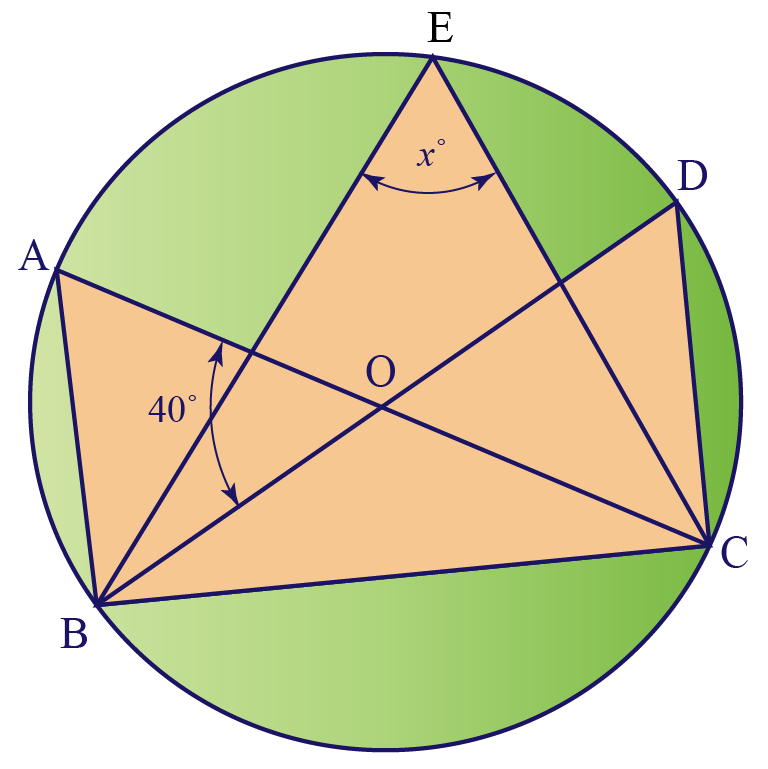Solution:

We have, $$\angle BOC=180^{\circ}-40^{\circ}=140^{\circ}$$

\implies x^{\circ}= \begin{align}\frac{1}{2}\end{align} \angle BOC=70^{\circ}

 $$\therefore$$ The value of $$x$$ is 70

VI.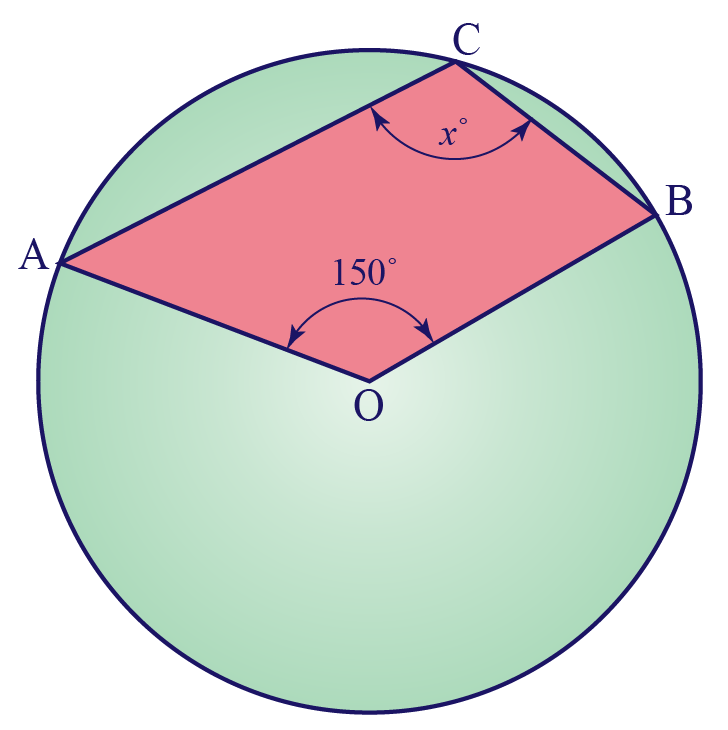Solution:

The reflex angle $$AOB$$ is equal to $$360^{\circ}-150^{\circ}$$ or $$210^{\circ}$$

Thus, x^{\circ}= \begin{align}\frac{1}{2}\end{align} \times 210^{\circ}=105^{\circ}

 $$\therefore$$ The value of $$x$$ is 105
 Example 2

In the following figure, find $$x^{\circ}$$ in terms of $$y^{\circ}$$ and $$z^{\circ}$$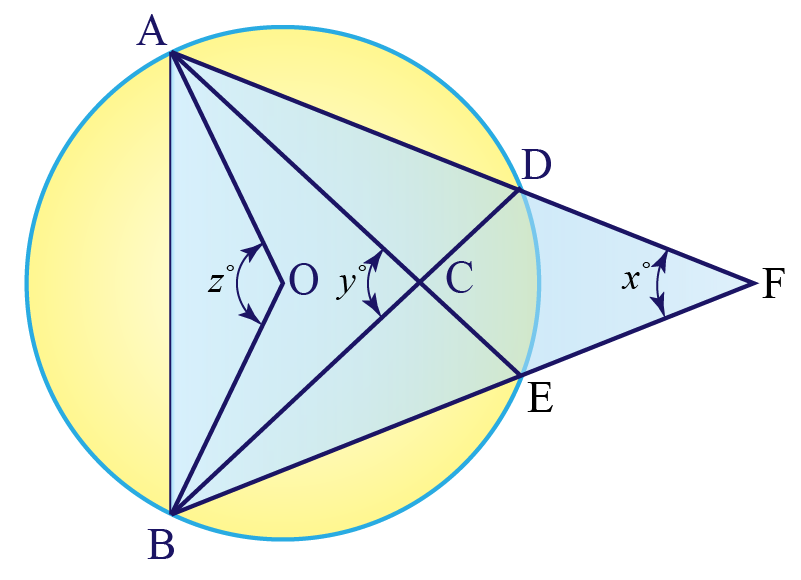$$O$$ is the centre of the circle.

Solution:

We label some angles as shown in the figure below.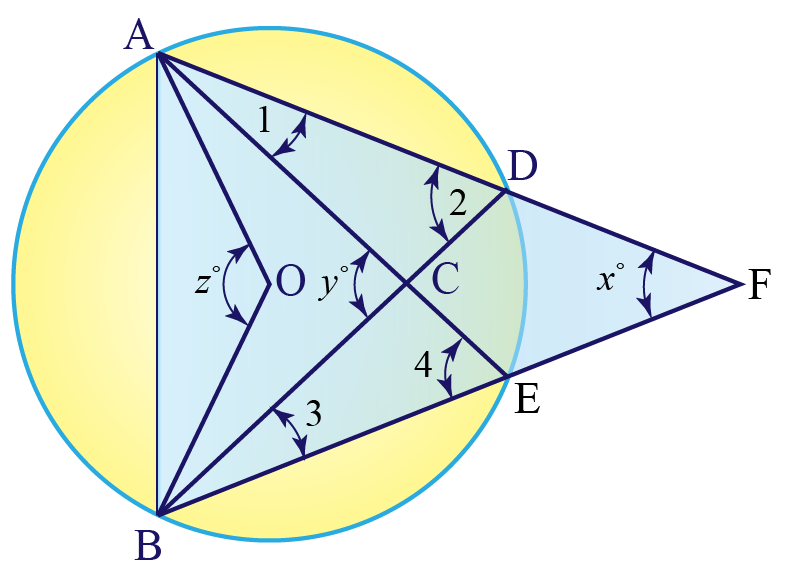Using the exterior angle theorem, we have,

$$\angle y = \angle 1 + \angle 2 \;\;\;\; \cdots (1)$$

$$\angle 1 + \angle x = \angle 4 \;\;\;\; \cdots (2)$$

Add Equations $$(1)$$ and $$(2$$

$$\angle y + \angle 1 + \angle x = \angle 1 + \angle 2 + \angle 4$$

$$\implies \angle x + \angle y = \angle 2 + \angle 4$$

Now, since $$z^{\circ} = 2 \angle 2 =2 \angle 4$$, we have \angle 2 = \angle 4 = \begin{align}\frac{z^{\circ}}{2}\end{align}

So,

\begin{align}x^{\circ}+y^{\circ}&=z^{\circ}\\ x^{\circ}&=z^{\circ}-y^{\circ}\end{align}

 $$\therefore$$ $$x^{\circ}=z^{\circ}-y^{\circ}$$
 Example 3

Two circles intersect at $$P$$ and $$Q$$.

Through $$P$$, diameters are drawn for both the circles.

The other end-points of these diameters are $$R$$ and $$S$$.

Show that $$R$$, $$Q$$ and $$S$$ are collinear.

Solution:

Consider the following figure.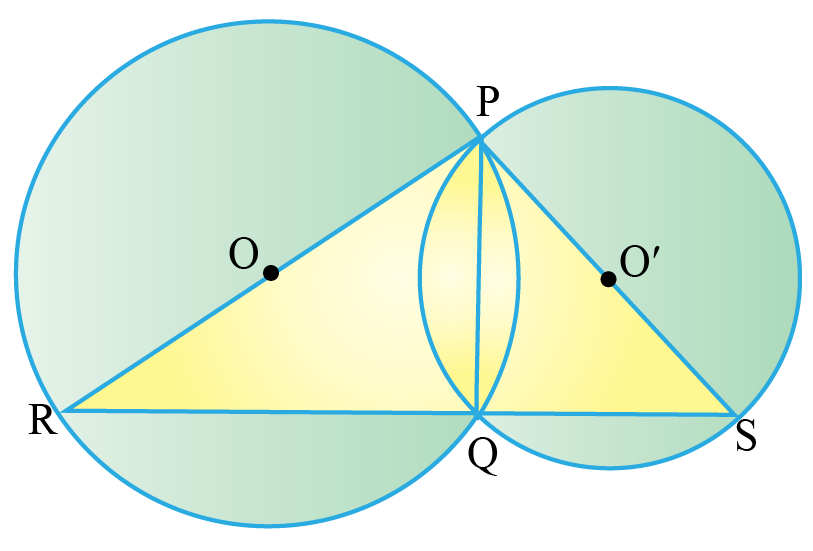Since angles in semi-circles are $$90^{\circ}$$, we have, $$\angle RQP=\angle SQP=90^{\circ}$$

Then,

\begin{align}\angle RQS&=\angle RQP+\angle SQP\\&=90^{\circ}+90^{\circ}\\&=180^{\circ}\end{align}

Thus, $$RQS$$ is a straight line, which means that $$R$$, $$Q$$ and $$S$$ collinear.

 $$\therefore$$ The points $$R$$, $$Q$$ and $$S$$ are collinear.
 Example 4

$$\Delta ABC$$ is isosceles, with vertex $$A$$

Show that the circle through diameter $$AB$$ bisects $$BC$$ at point $$D$$

Solution:

Observe the following figure.

The circle cuts $$BC$$ at $$D$$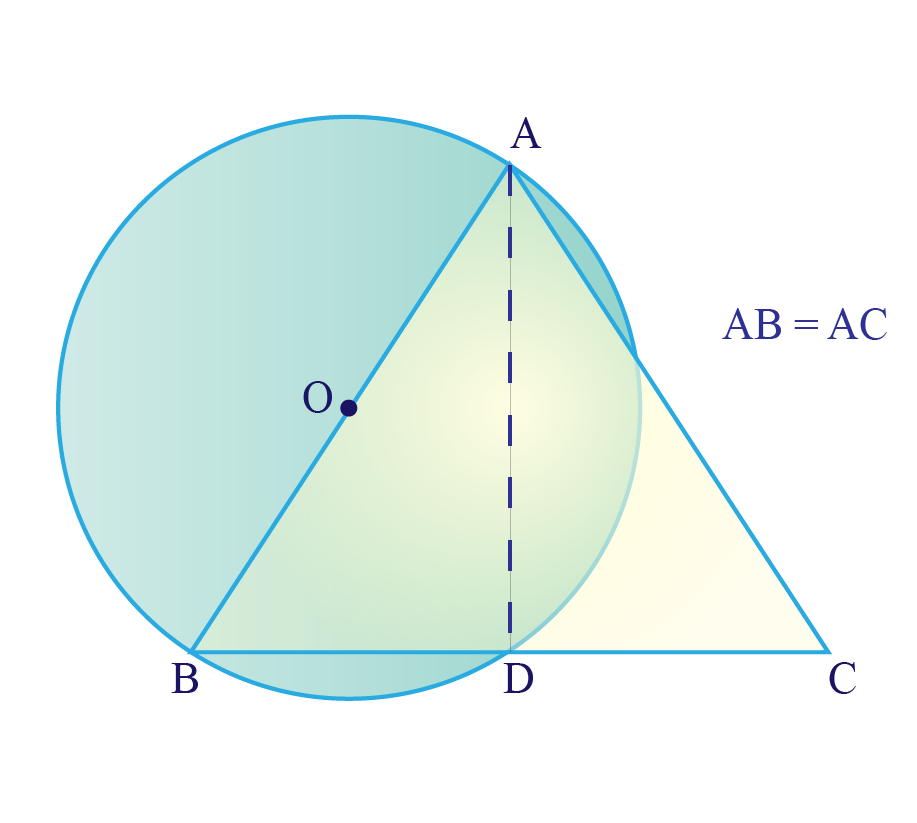Since angles in a semi-circle are of measure $$90^{\circ}$$, $$\angle ADB=90^{\circ}$$

This means $$AD$$ is the altitude for the isosceles $$\Delta ABC$$

$$\implies$$ $$AD$$ also bisects $$BC$$

 $$\implies$$ $$D$$ is the mid-point of $$BC$$
 Example 5

From the end-points of a diameter $$AB$$ of a circle with centre $$O$$, perpendiculars $$AP$$ and $$BQ$$ are dropped on an external line.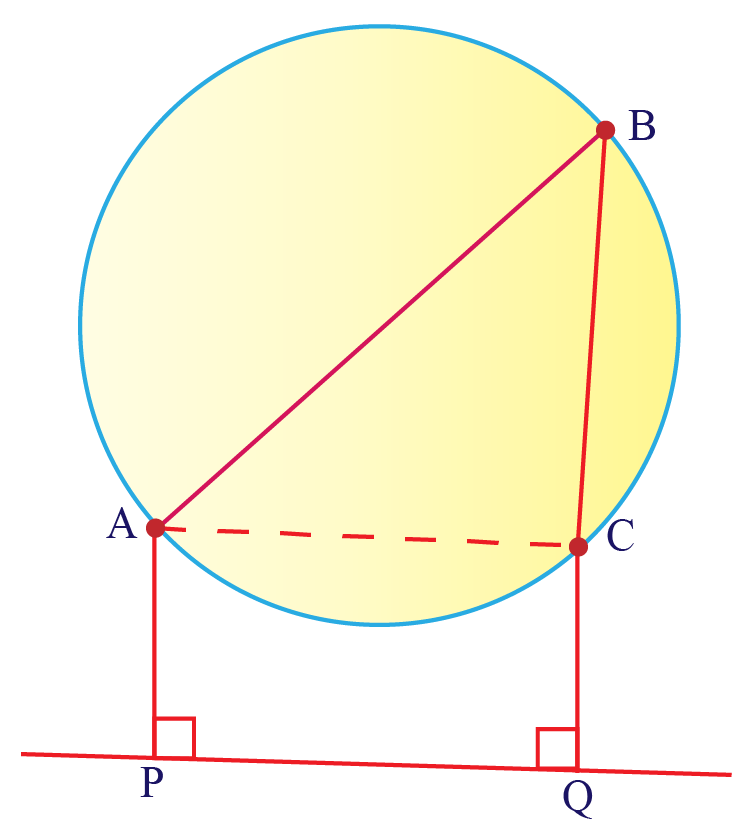Show that $$AP = CQ$$

Solution:

We note that $$\angle ACB = 90^{\circ}$$

Thus, $$ACQP$$ is a rectangle.

Since oppoite sides of a rectangle are equal, $$AP = CQ$$

 Hence, proved that $$AP = CQ$$